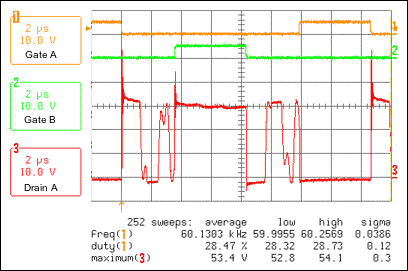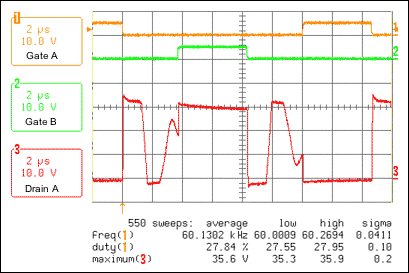# CCFL Push-Pull Snubber Circuit

### Abstract

The DS3984, DS3988, DS3881, DS3882, DS3992, and DS3994 are cold-cathode fluorescent lamp (CCFL) controllers that use a push-pull architecture to create the high-voltage AC waveforms needed to drive the lamps. In a push-pull drive scheme, the parasitic inductance of the step-up transformer, together with the parasitic capacitance of the output of the n-channel power MOSFETs, form a resonant circuit that can create unwanted voltage spikes. High-voltage spikes can increase the stress on the power MOSFETs and can also increase the electromagnetic interference (EMI) created by the system. This application note describes how to suppress the voltage spikes with a simple resistor-capacitor (RC) network.

### Unsuppressed Drain Voltages

Figure 1 details the typical gate drive and drain waveforms for push-pull drive scheme with a 15V DC supply. In a push-pull drive arrangement when the complimentary MOSFET is turned on, the drain voltage normally increases to twice the DC supply voltage (or 30V in this example). However, as shown in Figure 1, voltage spikes as high as 54V appear. Voltage spikes appear on the drain of the n-channel power MOSFET when the gate of the MOSFET is turned off and also when the gate of the complimentary MOSFET is turned on.### Circuit and Procedure for Suppressing the Drain Voltage Spikes

Suppressing the drain-voltage spikes can be accomplished with a simple RC network added to each drain, as shown in Figure 2. The proper values for the resistor (R) and capacitor (C) network can be determined from the following suppression procedure. An example that shows how to reduce the voltage spikes shown in Figure 1 is detailed after the procedure.### Procedure for Determining the Proper RC Values for the Snubber Circuit

1. Measure the frequency of the spike resonance. See Figure 3 for an example.
2. Add a shunt capacitor across the drain-source of the MOSFET (not the resistor, just the capacitor) and adjust the value of this capacitor until the frequency of the spike resonance is reduced by a factor of two. The value of this resulting capacitor will be three times the value of the parasitic capacitance that is creating the voltage spikes.
3. Because the parasitic capacitance is known, the parasitic inductance can be determined using the formula:

4. L = 1 / [(2πF)² x C] where F = resonant frequency and C = parasitic capacitance

5. Now that both the parasitic capacitance and inductance are known, the characteristic impedance of the resonance can be determined using the following formula:

6. Z = SQRT(L/C) where L = parasitic inductance and C = parasitic capacitance

7. The resistor in the RC snubber circuit should be sized to the value of the characteristic impedance, and the capacitor should be sized between four and ten times the parasitic capacitance. The use of larger capacitors slightly reduces the voltage overshoot at the expense of greater power dissipation and less inverter efficiency.

### Example of How to Determine the Proper RC Snubber Values

In this section, using the five-step procedure outlined previously, the proper values for the resistor (R) and capacitor (C) snubber circuit to reduce the Figure 1 voltage spikes are determined.

1. Find the frequency of the resonant spike voltage. Figure 3 shows it to be approximately 35MHz.
2.3. Apply a shunt capacitor across the drain to ground to reduce the resonant frequency to approximately half or 17.5MHz. As shown in Figure 4, a 330pF shunt capacitor reduces the resonant frequency to about 17.5MHz. The proper capacitor value was determined by trying different values of shunt capacitance. It is best to start with a small value (like 100pF) and proceed from there.
4. Because a 330pF shunt capacitor reduces the resonant frequency by a factor of two, the parasitic capacitance must be one-third of this value or about 110pF.5. Calculate the parasitic inductance.

6. Parasitic inductance = L = 1 / [(2 x 3.14 x 35MHz)² * 110pF] = 0.188µH

7. Calculate the characteristic impedance.

8. Characteristic impedance = Z = SQRT (0.188µH / 110pF) = 41

9. Select the proper values for the resistor and capacitor. The R in the snubber circuit should be close to 41Ω, and the C should be between four and ten times the parasitic capacitance of 110pF. In this example, we have chosen C to be 1000pF, which is about nine times the parasitic capacitance.

Figure 5 shows the result of adding a snubber circuit with an R of 39Ω and a C of 1000pF.### Conclusion

This application note has demonstrated that a few simple empirical measurements can be made to determine the proper values for a resistor-capacitor snubber circuit that significantly reduces the unwanted voltage spikes that can appear on the drains of the power MOSFETs in a push-pull drive arrangement.